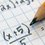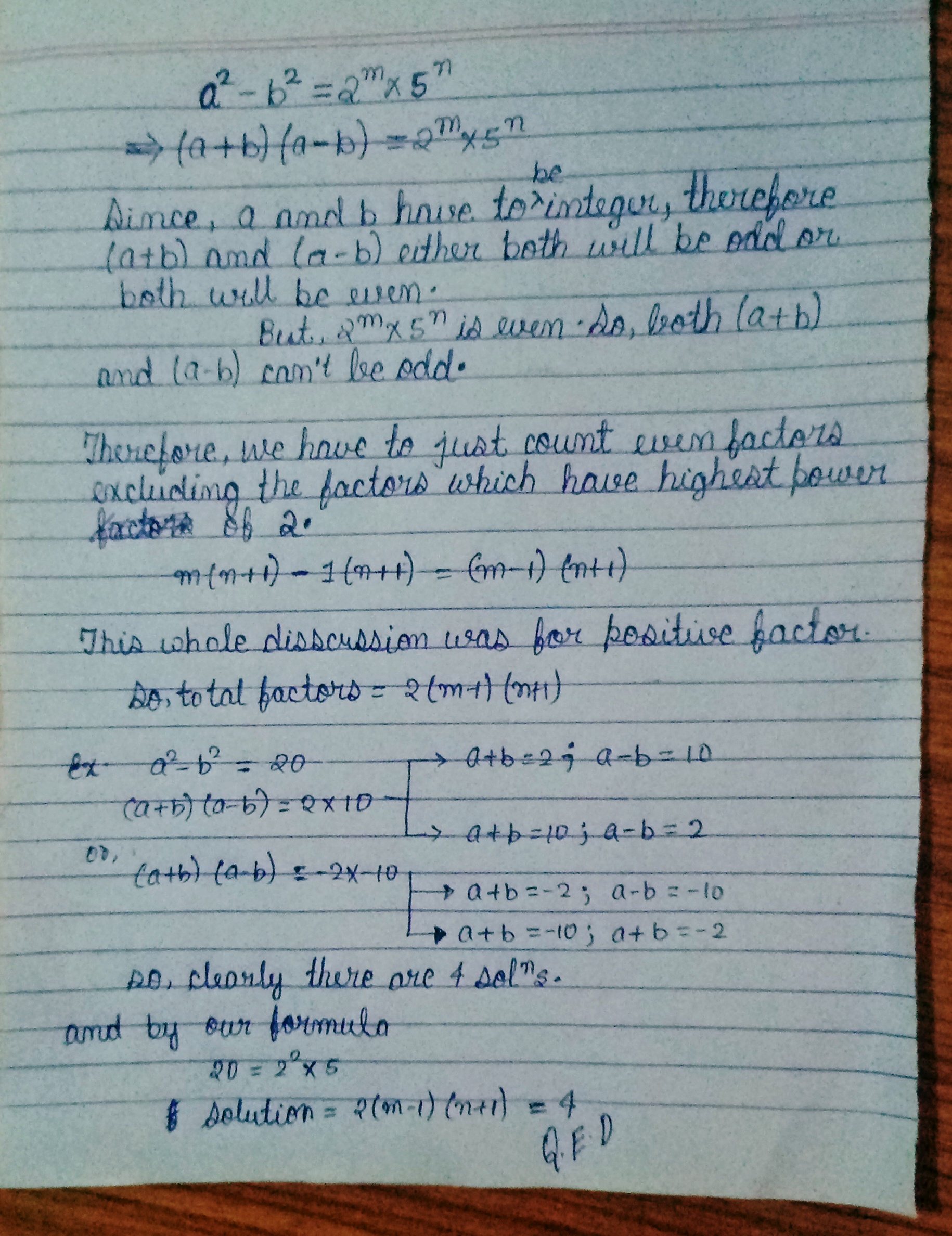# Integers $a,b$

How many pairs of integers $(a,b)$ satisfy $a^2-b^2=2000^2?$Note by Sudipto Podder
3 months ago

This discussion board is a place to discuss our Daily Challenges and the math and science related to those challenges. Explanations are more than just a solution — they should explain the steps and thinking strategies that you used to obtain the solution. Comments should further the discussion of math and science.

When posting on Brilliant:

• Use the emojis to react to an explanation, whether you're congratulating a job well done , or just really confused .
• Ask specific questions about the challenge or the steps in somebody's explanation. Well-posed questions can add a lot to the discussion, but posting "I don't understand!" doesn't help anyone.
• Try to contribute something new to the discussion, whether it is an extension, generalization or other idea related to the challenge.

MarkdownAppears as
*italics* or _italics_ italics
**bold** or __bold__ bold
- bulleted- list
• bulleted
• list
1. numbered2. list
1. numbered
2. list
Note: you must add a full line of space before and after lists for them to show up correctly
paragraph 1paragraph 2

paragraph 1

paragraph 2

[example link](https://brilliant.org)example link
> This is a quote
This is a quote
    # I indented these lines
# 4 spaces, and now they show
# up as a code block.

print "hello world"
# I indented these lines
# 4 spaces, and now they show
# up as a code block.

print "hello world"
MathAppears as
Remember to wrap math in $$ ... $$ or $ ... $ to ensure proper formatting.
2 \times 3 $2 \times 3$
2^{34} $2^{34}$
a_{i-1} $a_{i-1}$
\frac{2}{3} $\frac{2}{3}$
\sqrt{2} $\sqrt{2}$
\sum_{i=1}^3 $\sum_{i=1}^3$
\sin \theta $\sin \theta$
\boxed{123} $\boxed{123}$

Sort by:

@Sudipto Podder, i have checked my formula for different values from wolframalpha.

- 2 months, 3 weeks ago

👍🏻

- 2 months, 3 weeks agoHere is my process bro.

- 2 months, 4 weeks ago

Thanks

- 2 months, 4 weeks ago

Welcome!

- 2 months, 3 weeks ago

You can apply this formula on your question as well. I have seen that your thought process was similar.

- 2 months, 4 weeks ago

Look my thought was something like: a²-b²=2000² Or, (a+b)(a-b)= 2000² Now , we have to express 2000² like such k×m where we can say that (a+b)=k and (a-b)=m ...and when we use addition in those equations , we get 2a =k+m ....but then .. a can be an integer if (k+m) is an even number....in that case k and m should be in the same parity ...... Now 2000²=(2^8)×(5^6) Here both k and m can't be odd.... because there should be 2 as a factor in at least one of k and m........so both k and m should be even...now you can express (2^8)×(5^6) as k×m in total {(8+1)×(6+1)}=63 ways . But in both k and m, there should be both 2 and 5 as factors.... otherwise one of them will definitely be odd .....here in those 63 ways , there are {(6+1)×2}=14 ways where there are one odd and one even between k and m.....so total positive ways of expressing 2000² as k×m( following all the conditions) are of (63-14)=49 types...... But here you have to notice that all these discussions are for positive integers...but it is also possible for negative integers ...so the total ways are of (49×2)=98 types SO, THE ANSWER IS [{(98)}]

- 2 months, 4 weeks ago

Your intution is correct,but i think you don't know how to calculate even factors.See my answer.

- 2 months, 4 weeks ago